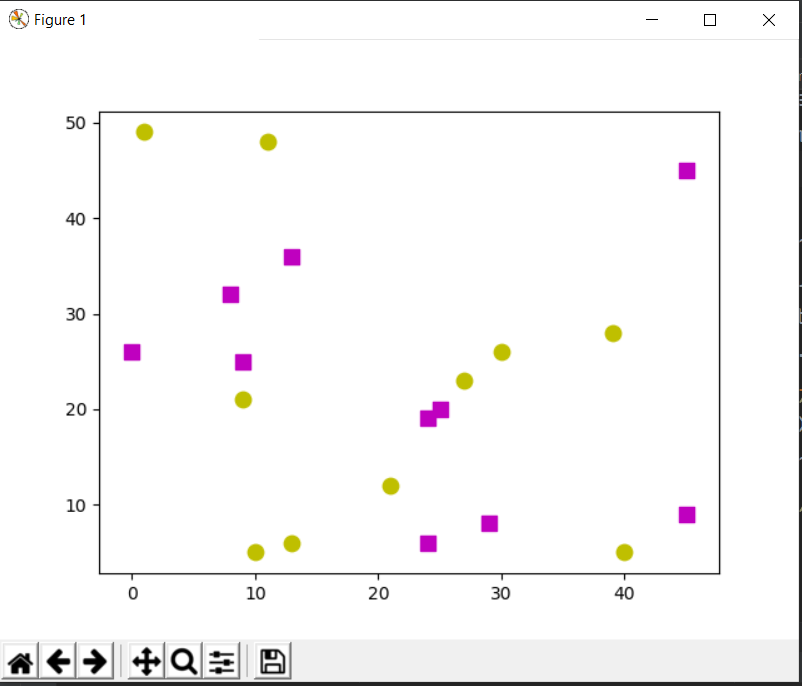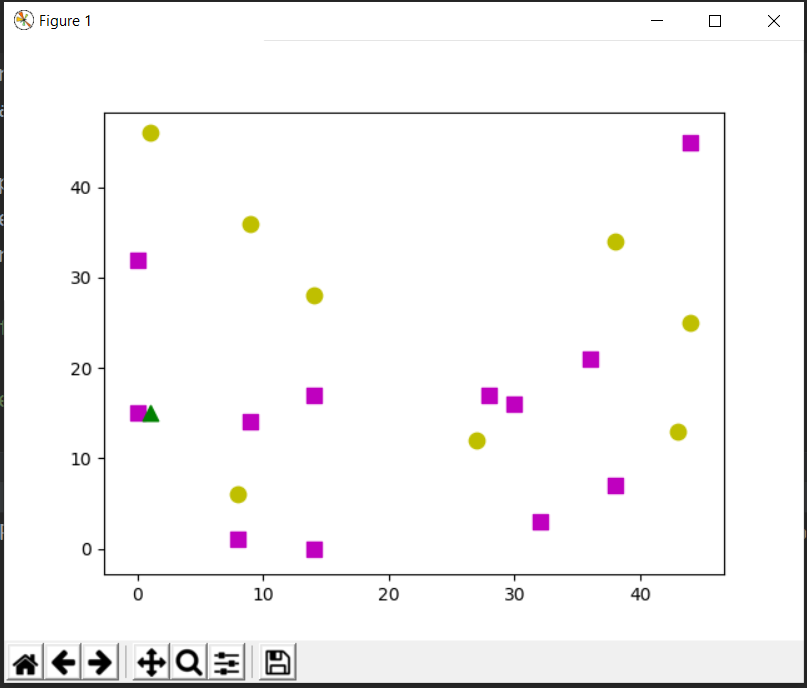# Implementation of KNN using OpenCV

KNN is one of the most widely used classification algorithms that is used in machine learning. To know more about the KNN algorithm read here KNN algorithm

Today we are going to see how we can implement this algorithm in OpenCV and how we can visualize the results in 2D plane showing different features of classes we have in our training data.

Let’s consider two classes for our code. We generate 20 random data points belonging to the 2 classes using a random generator. The training points will be either of the ‘magenta’ class or ‘yellow’ class. The magenta is drawn as square and the label for magenta is 1 similarly yellow is drawn as a circle and is labelled as 0.

Code:

 `# Import necessary libraries ` `import` `cv2 as cv ` `import` `numpy as np ` `import` `matplotlib.pyplot as plt ` ` `  `# Create 20 data points randomly on the 2-D plane. ` `# Data_points are random points located on the 2D plane. ` `Data_points ``=` `np.random.randint(``0``, ``50``, (``20``, ``2``)).astype(np.float32) ` ` `  `# Label the data points with their class labels. ` `labels ``=` `np.random.randint(``0``, ``2``, (``20``, ``1``)).astype(np.float32) ` `# labels are the classes assigned to data points. ` ` `  `# Take the yellow class for 0 label and magenta class for 1 label ` `yellow ``=` `Data_points[labels.ravel()``=``=` `0``] ` `magenta ``=` `Data_points[labels.ravel()``=``=` `1``] ` ` `  `# Plot the classes on the 2-D plane ` `# o for circle ` `plt.scatter(yellow[:, ``0``], yellow[:, ``1``], ``80``, ``'y'``, ``'o'``)  ` `# s for sqaure ` `plt.scatter(magenta[:, ``0``], magenta[:, ``1``], ``80``, ``'m'``, ``'s'``) ` `plt.show() `

Output:Now consider an unknown new data point, our KNN classifier will label that data point either 0 or 1 depending on its features and the number of neighbours that are defined by us.
Code:

 `# generate a random data point ` `# unkown is a random data point for which we will perform prediction. ` `unknown ``=` `np.random.randint(``0``, ``50``, (``1``, ``2``)).astype(np.float32) ` `# create the knn classifier ` `knn ``=` `cv.ml.KNearest_create() ` ` `  `# we use cv.ml.ROW_SAMPLE to occupy a row of samples from the samples. ` `knn.train(Data_points, cv.ml.ROW_SAMPLE, labels) ` `# get the labelled result, the numbers, the distance of each data point. ` `# find nearest finds the specified number of neighbours and predicts responses. ` `ret, res, neighbours, distance ``=` `knn.findNearest(unknown, ``5``) ` ` `  `# For each row of samples the method finds the k nearest neighbours.  ` `# For regression problems, the predicted result is a mean of all the neighbours.  ` `# For classification, the class is determined by the majority. ` ` `  `# plot the data point with other data points ` `plt.scatter(unknown[:, ``0``], unknown[:, ``1``], ``80``, ``'g'``, ``'^'``) ` `# show the result. ` `plt.show() ` ` `  `# print the results obtained ` `print``( ``"Label of the unknown data - "``, res ) ` `print``( ``"Nearest neighbors -  "``, neighbours ) ` `print``( ``"Distance of each neighbor - "``, distance ) `

Output:

```Label of the unknown data -  [[1.]]
Nearest neighbors -   [[1. 1. 0. 1. 1.]]
Distance of each neighbor -  [[  1.  65. 130. 173. 245.]]```My Personal Notes arrow_drop_upCheck out this Author's contributed articles.

If you like GeeksforGeeks and would like to contribute, you can also write an article using contribute.geeksforgeeks.org or mail your article to contribute@geeksforgeeks.org. See your article appearing on the GeeksforGeeks main page and help other Geeks.

Please Improve this article if you find anything incorrect by clicking on the "Improve Article" button below.

Article Tags :
Practice Tags :

Be the First to upvote.

Please write to us at contribute@geeksforgeeks.org to report any issue with the above content.